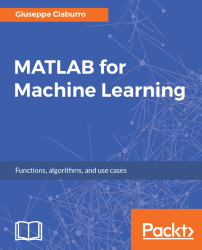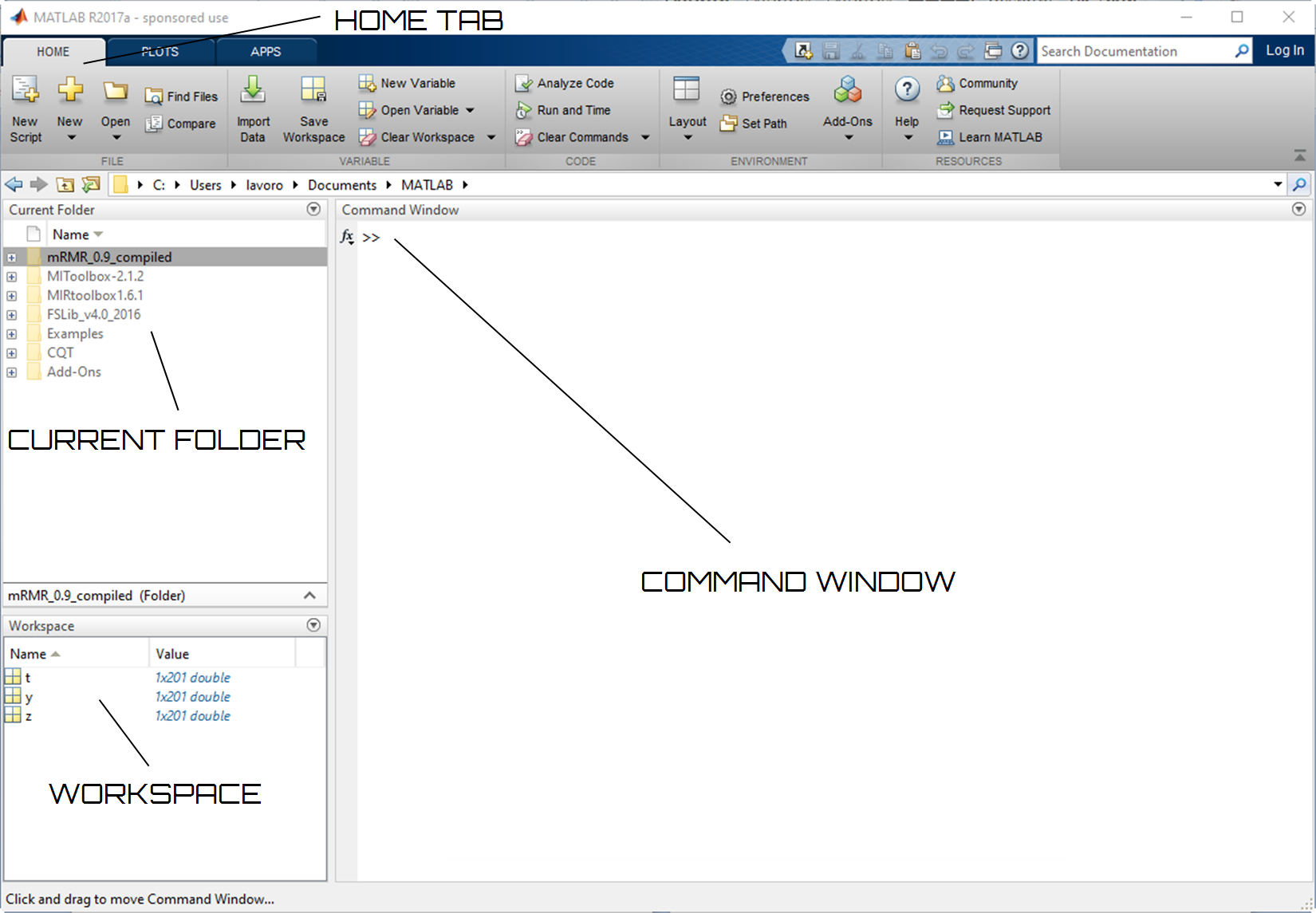•#### MATLAB for Machine Learning#### Overview of this book

MATLAB is the language of choice for many researchers and mathematics experts for machine learning. This book will help you build a foundation in machine learning using MATLAB for beginners. You’ll start by getting your system ready with t he MATLAB environment for machine learning and you’ll see how to easily interact with the Matlab workspace. We’ll then move on to data cleansing, mining and analyzing various data types in machine learning and you’ll see how to display data values on a plot. Next, you’ll get to know about the different types of regression techniques and how to apply them to your data using the MATLAB functions. You’ll understand the basic concepts of neural networks and perform data fitting, pattern recognition, and clustering analysis. Finally, you’ll explore feature selection and extraction techniques for dimensionality reduction for performance improvement. At the end of the book, you will learn to put it all together into real-world cases covering major machine learning algorithms and be comfortable in performing machine learning with MATLAB.
Title PageCreditsForewordwww.PacktPub.comCustomer FeedbackPrefaceFree Chapter
Getting Started with MATLAB Machine LearningImporting and Organizing Data in MATLABFrom Data to Knowledge DiscoveryFinding Relationships between Variables - Regression TechniquesPattern Recognition through Classification AlgorithmsIdentifying Groups of Data Using Clustering MethodsSimulation of Human Thinking - Artificial Neural NetworksImproving the Performance of the Machine Learning Model - Dimensionality ReductionMachine Learning in Practice## Familiarizing yourself with the MATLAB desktop

MATLAB is an interactive working environment based on the matrix, the most natural way to express computational mathematics. Moreover, it is a programming language designed for technical computing, mathematical analysis, and system simulation. The integrated graphics make it easy to view and acquire knowledge from the data. Finally, a large library of prebuilt toolboxes allows us to immediately start with programming.

After installing, to start MATLAB, simply double-click on the icon on the desktop; or from the system prompt, type `matlab`. At first startup, MATLAB will show us a desk of contents with all the necessary items for its proper and smooth operation. Figure 2.1 shows the MATLAB desktop in the R2017a version:Figure 2.1: MATLAB R2017a desktop for Windows 10

The MATLAB desktop includes the following panels:

• `Current Folder`: Shows the files that are stored in this folder (we can change it)
• `Command Window`: At the prompt (`>>`), any MATLAB...